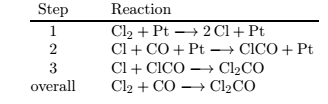Chemistry Practice Problems Reaction Mechanism Practice Problems Solution: Consider the reaction mechanism given below. Whic...

# Solution: Consider the reaction mechanism given below. Which species is/are intermediates? 1. Cl, ClCO 2. ClCO 3. Pt, ClCO 4. Pt 5. Cl 6. Pt, Cl

###### Problem

Consider the reaction mechanism given below.

Which species is/are intermediates?

1. Cl, ClCO

2. ClCO

3. Pt, ClCO

4. Pt

5. Cl

6. Pt, ClReaction Mechanism

Reaction Mechanism

#### Q. Consider the following reaction mechanism Ce4+ (aq) + Mn2+ (aq) ↔ Ce3+(aq) + Mn3+ (aq) Ce4+ (aq) Mn3+ (aq) ↔ Ce3+ (aq) + Mn4+ (aq) Ti+ (aq) + Mn4+ ...

Solved • Thu Aug 25 2016 11:47:35 GMT-0400 (EDT)

Reaction Mechanism

#### Q. Consider the reaction: 2 AB → C2 + 2 BC The following mechanism has been proposed. 2 AB → A2B2                       slow second step             ...

Solved • Thu Aug 25 2016 09:14:37 GMT-0400 (EDT)

Reaction Mechanism

#### Q. Given the following proposed mechanism, predict the rate law for the overall reaction.A2 + 2B → 2AB (overall reaction)MechanismA2 ⇌ 2A     fastA + B  ...

Solved • Wed Aug 24 2016 20:50:27 GMT-0400 (EDT)

Reaction Mechanism

#### Q. The oxidation of iron (II) ions by iodine in aqueous solution occurs as shown below2 Fe2+ (aq) + l2 (aq) → 2 Fe3+ (aq) + 2 l - (aq)and the experimenta...

Solved • Wed Aug 24 2016 17:38:35 GMT-0400 (EDT)